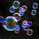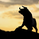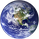‎45200‎ مشاهدة
45200
These Bollinger bands feature Fibonacci retracements to very clearly show areas of support and resistance . The basis is calculate off of the Volume Weighted Moving Average . The Bands are 3 standard deviations away from the mean. 99.73% of observations should be in this range.
```study(shorttitle="FBB", title="Fibonacci Bollinger Bands", overlay=true)
length = input(200, minval=1)
src = input(hlc3, title="Source")
mult = input(3.0, minval=0.001, maxval=50)
basis = vwma(src, length)
dev = mult * stdev(src, length)
upper_1= basis + (0.236*dev)
upper_2= basis + (0.382*dev)
upper_3= basis + (0.5*dev)
upper_4= basis + (0.618*dev)
upper_5= basis + (0.764*dev)
upper_6= basis + (1*dev)
lower_1= basis - (0.236*dev)
lower_2= basis - (0.382*dev)
lower_3= basis - (0.5*dev)
lower_4= basis - (0.618*dev)
lower_5= basis - (0.764*dev)
lower_6= basis - (1*dev)
plot(basis, color=fuchsia, linewidth=2)
p1 = plot(upper_1, color=white, linewidth=1, title="0.236")
p2 = plot(upper_2, color=white, linewidth=1, title="0.382")
p3 = plot(upper_3, color=white, linewidth=1, title="0.5")
p4 = plot(upper_4, color=white, linewidth=1, title="0.618")
p5 = plot(upper_5, color=white, linewidth=1, title="0.764")
p6 = plot(upper_6, color=red, linewidth=2, title="1")
p13 = plot(lower_1, color=white, linewidth=1, title="0.236")
p14 = plot(lower_2, color=white, linewidth=1, title="0.382")
p15 = plot(lower_3, color=white, linewidth=1, title="0.5")
p16 = plot(lower_4, color=white, linewidth=1, title="0.618")
p17 = plot(lower_5, color=white, linewidth=1, title="0.764")
p18 = plot(lower_6, color=green, linewidth=2, title="1")```
تمت إزالة التعليقI will repurpose this for my own use....thanks for a good start.
ردhi this is wrong
i apply this script but this is not correct
but very good idea
ردEK_Analysis
@EK_Analysis, what exactly is "incorrect"? Remember Technical Analysis is an not a precise science. An indicator need not be 100% accurate to generate substantial profits :)
ردkourosht
@kourosht, A good model should be able to be applied to many of types of assets. Like such, this indicator is can be used for many different types of assets.
رد